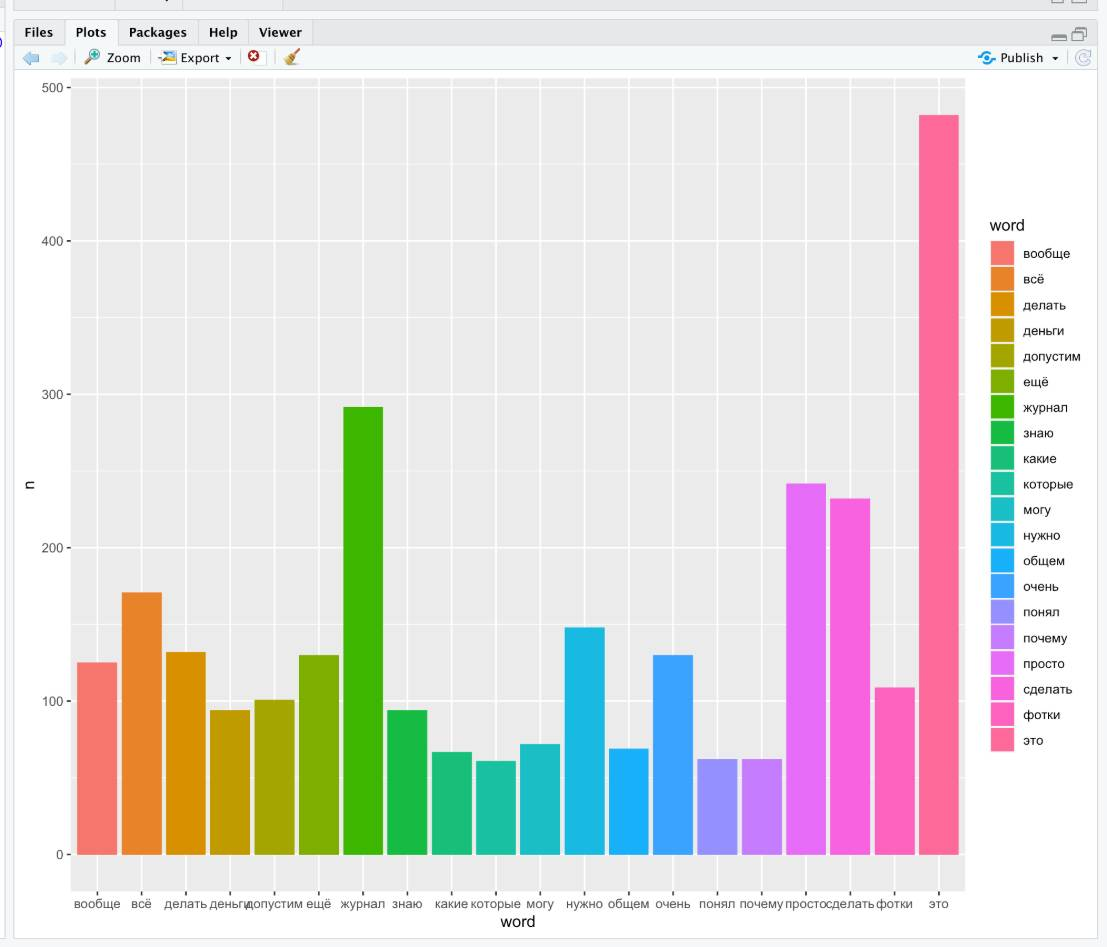# R : word frequency in dataframe

Alright so in the short tutorial we’ll calculate word frequency and visualize it.

BUT when it comes for stopwords and language different from English, there might be some difficulties.

I’ve a dataframe which has field text is russian language.

## Step 0 : Install required libraries

``````packages.install("tidyverse")
packages.install("tidytext")
packages.install("tm")
library(tidyverse)
library(tidytext)
library(tm)
``````

## Step 1 : Create stopwords dataframe

``````#create stopwords DF
rus_stopwords = data.frame(word = stopwords("ru"))
``````

## Step 2 : Tokenize

``````new_df <- video %>% unnest_tokens(word, text) %>% anti_join(rus_stopwords)

# - anti_join  - functoin to remove stopwords
#video - is name of dataframe
#word - is name of new field
#text - is just a filed with our text
``````

## Step 3 : Count words

``````frequency_dataframe = new_df %>% count(word) %>% arrange(desc(n))
``````

## Step 4 (Optional) : Take only first 20 items from a dataframe

``````short_dataframe = head(frequency_dataframe, 20)
``````

## Step 5 Visualize with ggplot

``````ggplot(short_dataframe, aes(x = word, y = n, fill = word)) + geom_col()
``````

So in my case it looked looked like this: